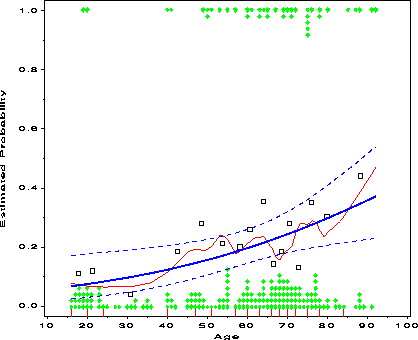logodds Plot empirical log-odds for logistic regression logodds

# Visualizing Categorical Data: logodds

\$Version: 1.1 (11 Mar 2001)
Michael Friendly
York University

## The logodds macro (get logodds.sas)

### Plot empirical log-odds for logistic regression

For a binary response variable, Y, taking values 0 or 1, and a continuous independent variable, X, the LOGODDS macro groups the X variable into some number of ordered, non-overlapping intervals. It plots the empirical log-odds of `Y=1` (and/or Pr{`Y=1`}) against X for each interval of X, together with the fitted linear logistic relation, an optional smoothed curve (using the LOWESS macro), and the observed binary responses.

## Usage

The input data to be plotted must be in case form. The LOGODDS macro is called with keyword parameters. The X= and Y= variables are required. The arguments may be listed within parentheses in any order, separated by commas. For example:
```
%logodds(data=icu, x=age, y=died);
```

### Parameters

X=
Name of the continuous independent variable
Y=
Name of the binary response variable
EVENT=
Value of Y for the event of interest [Default: `EVENT=1`]
DATA=
The name of the input data set [Default: `DATA=_LAST_`]
OPTIONS=
Options for PROC LOGISTIC, for example, `OPTIONS=DESCENDING`.
NCAT=
Number of categories of the X variable. For example, if deciles of X are desired, use `NCAT=10`. [Default: `NCAT=10`]
PLOT=
`Scale(s)` for the response. `PLOT=LOGIT` gives a plot on the logit scale, `PLOT=PROB` on the probability scale. [Default: `PLOT=LOGIT PROB`]
SMOOTH=
Smoothing parameter for a lowess smooth, in the interval (0-1). No smooth curve is produced unless a SMOOTH= value is specified.
SHOW=
Specifies whether to plot the binary observations. [Default: `SHOW=OBS`]
OBS=
Specifies how to display the binary observations. If `OBS=STACK`, the observations are plotted in vertical columns at the top (`Y=1`) or bottom (`Y=0`) of the plot. If `OBS=JITTER` a small random quantity is added (`Y=0`) or subtracted (`Y=1`) to the Y value. [Default: `OBS=STACK`]
NAME=
The name of the graph in the graphic catalog [Default: `NAME=LOGODDS`]
GOUT=
The name of the graphic catalog [Default: `GOUT=GSEG`]

### Example

```
%include catdata(icu);
%logodds(data=icu, x=age, y=died, smooth=0.25, ncat=16,
options=order=data);
```